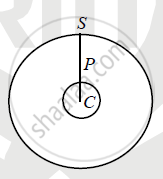Share

# Derive an Expression for Acceleration Due to Gravity at Depth ‘D’ Below the Earth’S Surface. - Physics

#### Question

Derive an expression for acceleration due to gravity at depth ‘d’ below the earth’s surface.

#### Solution

Let M be mass of the earth, R be the radius of the earth

gd be gravitational acceleration at depth 'd ' from the earth surface

g be gravitational acceleration on the earth surfaces.

ρ be the density of the earth.‘P’ be the point inside the earth at depth 'd ' from earth surfaces.

∴ CS-CP=d, ∴ CP=R-d .............(1)   (since CS=R)

g=(GM)/R^2,

therefore g=(G4/3piR^3rho)/R^2

therefore g=(4GpiRrho)/3  ....(2)

gd = acceleration due to gravity at depth 'd '

g_d=(Gxx"Mass of the sphere with radius CP")/(CP^2)

thereforeg_d=(G4/3piCP^3rho)/(CP^2)

thereforeg_d=(4GpiCPrho)/3 .... (3)

Dividing eq. (3) by eq. (2)

g_d/g=(CP)/R=(R-d)/R

therefore g_d=g(1-d/R)

c

Is there an error in this question or solution?